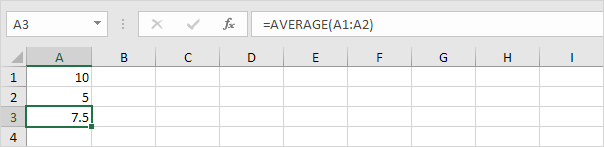# Formula Bar

The formula bar in Excel displays the value or formula of the active cell. You can use the formula bar to enter or edit formulas.

### Where is the Formula Bar?

The formula bar is located below the ribbon and above the worksheet area. To enter a formula, execute the following steps.

1. Select a cell.

2. To let Excel know that you want to enter a formula, type an equal sign (=).

3. For example, type the formula A1*A2 and press Enter.

Excel displays the result (50) in the cell and the formula in the formula bar.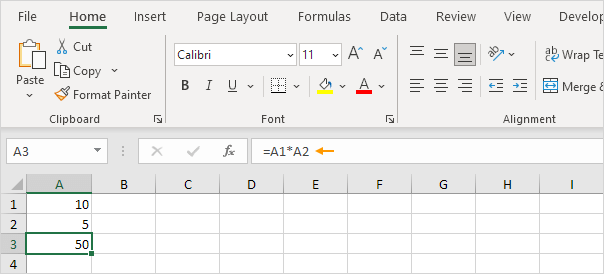Note: use the asterisk symbol (*) to multiply numbers in Excel.

### Edit a Formula

You can use the formula bar to quickly edit a formula in a cell (or to change the value in a cell).

1. Select a cell containing a formula.

2. Click in the formula bar and change the formula.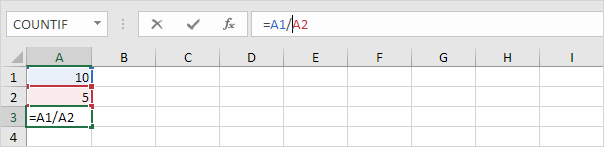Note: use the forward slash (/) to divide numbers in Excel.

3. Press Enter.### Show Formula Bar

Show the formula bar so you can see formulas in cells. If your formula bar is missing, execute the following steps.

1. On the View tab, in the Show group, check the Formula Bar box.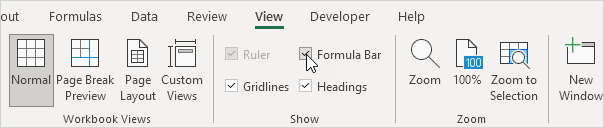Note: to hide the formula bar, uncheck the Formula Bar box. Sometimes this can be useful if you want to view more of your worksheet area.

### Expand Formula Bar

When you have a very long formula (for example, a nested IF formula), you can always expand the formula bar.

1. Hover over the bottom of the formula bar. A two-headed arrow appears.

2. Click and hold the left mouse button.

3. Drag the bar down.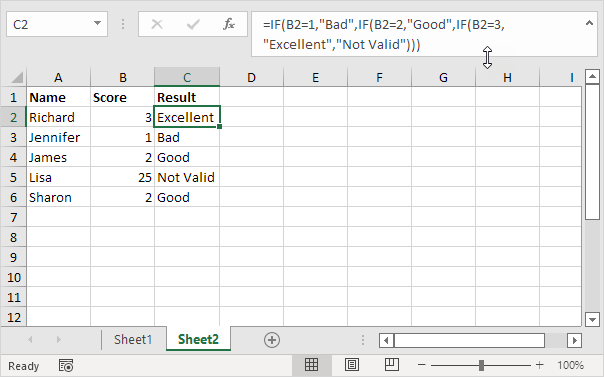### Insert Function

If you're new to functions in Excel, you can use the Insert Function button on the formula bar to search for a function.

1. First, select cell A3 and press Delete (on your keyboard) to delete the formula in cell A3.

2. Click the Insert Function button.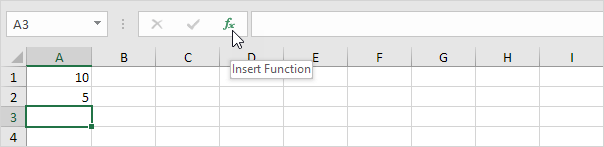Excel opens the Insert Function dialog box.

3. You can search for a function or browse functions by category.

4. Select a function and click OK.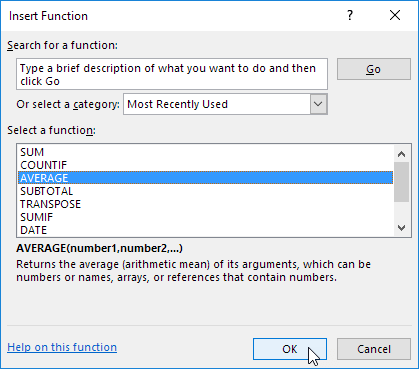Excel opens the Functions Arguments dialog box.

5. Excel automatically adds the range A1:A2 (the arguments or input passed to the function).

6. Click OK.Result.